# The Geometric Viewpoint

geometric and topological excursions by and for undergraduates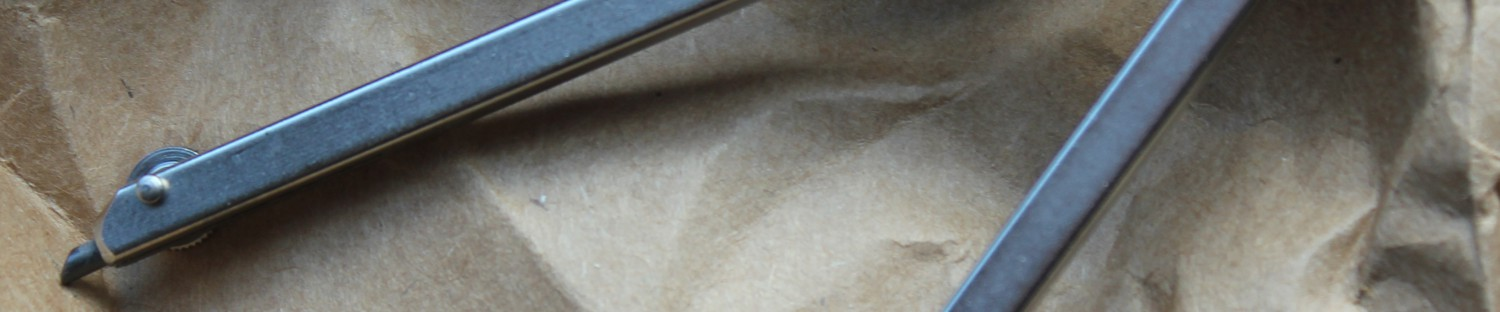• Monthly Archives December 2016
• ## Tessellations of the Hyperbolic Plane and M.C. Escher

(this post is by Allyson Redhunt)

“For me it remains an open question whether [this work] pertains to the realm of mathematics or to that of art.” -M. C. Escher

Escher’s beginnings
M. C. Escher (1898-1972) (Figure 1) is known for his mind-boggling artwork that challenges our sense of space. Although many of his works are artistic renditions of deep mathematical ideas, he had no formal training in mathematics. In fact, he was a poor student in high school–struggling to earn a diploma. In his post-secondary education, he was trained at the School of Architecture and Decorative Arts in Haarlem. This is where he developed his fascination with structure.
Although he began as an architecture student, he was soon switched to a decorative arts path. During his schooling, he did some traveling and was exposed to Moorish motifs. These works of design are created from mathematically careful patterns. From their influence, Escher’s art branched into tessellations of the plane. He was inspired by the idea of “approaches to infinity” and began by playing with flat surfaces and spheres.

Escher’s ideas about structure, pattern, and infinity were suddenly enhanced when he came across the work of geometer H. S. M. Coxeter (1907-2003). Coxeter and Escher struck up a correspondence when Coxeter hoped to use Escher’s unique depictions of symmetry in a presentation for the Royal Society of Canada. Coxeter sent Escher a copy of the talk, which included an illustration depicting a tessellation of the hyperbolic plane (Figure 3) . This image sparked a new area of Escher’s exploration of infinity . To fully understand the beauty of his works, it is helpful to have a basic understanding of hyperbolic geometry.

A crash course in hyperbolic geometry
So what is hyperbolic space? Grade school mathematics is taught using Euclidean geometry. This assumes Euclid’s axioms, which he intended to be the basis of all geometry. However, one of them was a great source of debate between mathematicians. The “Parallel Postulate,” which states that if one straight line crosses two other straight lines to make both angles on one side less than 90˚, then the two lines meet. Proving that triangles have 180˚ angle sums is an application of this postulate .
However, the Parallel Postulate need not hold true in all cases, such as on the surface of a sphere. Proving that the postulate need not hold led to the discovery of an important “non-Euclidean” geometry called hyperbolic geometry. Although it at first seems unnatural to think about parallel lines performing in “new” ways, hyperbolic surfaces can be found in nature. Two common examples are sea slugs (Figure 4) and lettuce (Figure 5). The wavy structure is the tip-off that their surfaces exhibit hyperbolic geometry. This is because, while a Euclidean surface has curvature equal to zero everywhere, a hyperbolic surface has constant negative curvature (for comparison, a sphere has constant positive curvature).Figure 4. Close up of a colorful nudibranch .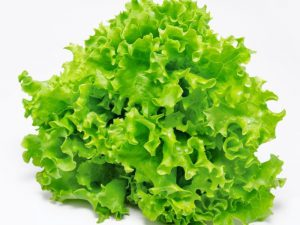Figure 5. Leafy lettuce .

Hyperbolic geometry has many interesting properties that counter our ingrained Euclidean intuition. To understand them, we will explore an important model of hyperbolic space: the Poincaré disc model.
The Poincaré disc model
We can imagine hyperbolic space as an open disk in the complex plane $\C$. We think of the space in the disk getting infinitely more “dense” as we approach the boundary of the disc, so the distance of a straight line between two points get longer as we approach the boundary. Thus, the shortest path between two points may be curved to take advantage of the “less dense” area towards the center of the disc. It turns out that the shortest distance between two points lies along the arc of a circle that is perpendicular to the boundary. In Figure 6, the shortest distance, called a geodesic, between A and B is the arc length of the given circle. Thus, the idea of “lines” in Euclidean space is generalized when in hyperbolic space to include circles.
This disc model is precisely what Escher saw in Coxeter’s book, and is what he used to create his art.

Polygons in hyperbolic space
Since lines in hyperbolic space differ from our intuition about lines in Euclidean space, we must adjust our understanding of polygons as well. A hyperbolic polygon is a region of the hyperbolic plane whose boundary is decomposed into finitely many generalized line segments (recall that this includes circle segments), called edges, meeting only at their endpoints, called vertices. At most two edges can meet at any one point .
Earlier we mentioned that Euclid’s axiom that fails in hyperbolic space is used to prove that triangles have an interior angle sum of 180˚. Since this axiom does not hold, we need a new framework for thinking about polygons in hyperbolic space. In particular, Euclidean rectangles–quadrilaterals having four 90˚ angles–do not exist in hyperbolic space.
The basis for the polygon framework in hyperbolic space is the Gauss-Bonnet formula, which tells us that the area of a convex geodesic n-gon is$(n-2)\pi$ minus the sum of the interior angles . Let’s take a moment for this to sink in–the area of polygons in hyperbolic space is dependent completely on angles! This goes against our Euclidean intuition, which tells us that a triangle with longer side lengths has a larger area than a triangle with shorter side lengths, despite the fact that both have angle sums of 180˚.
Armed with this knowledge that hyperbolic space gets more “dense” along with these new ideas about polygons, we are ready to dig in to the math of Escher’s works: hyperbolic tessellations.

Tessellations of hyperbolic space
Anyone who has looked at a tiled bathroom floor is familiar with the idea of tessellations: If we repeat a pattern of polygons, we can create a pattern over a large space. Someone who has attempted to tile their own bathroom floor may have noticed that not all tile shapes fit together nicely in a pattern. Note that a bathroom floor is an example of a Euclidean space, a geometry in which it turns out to be relatively difficult to happen upon a true tessellation. In contrast, hyperbolic space is relatively easy to tessellate.
Formally, a tessellation is a polygonal tiling of a plane that covers the entire plane. The Tessellation Theorem states that any polygon tiling in a complete space with the angles around any vertex adding to$\frac{2\pi}{n}$ for some integer n > 0 will be a tessellation of the plane. This holds true for Euclidean, spherical, and hyperbolic geometries.

When thinking about the increased “density” as we approach the edge, it should not be surprising that polygons appear smaller as we approach the boundary. Despite this, the areas stay constant (recall that it depends only on the angles!).

Escher’s tessellations
Escher created five works inspired by hyperbolic plane tessellations: Circle Limits I-IV and Snakes. While Circle Limit II and Snakes are beautiful (I highly recommend looking them up), in them Escher took more artistic license in tessellating the hyperbolic plane than the others. Thus, we will investigate Circle Limits I, III, and IV, all of which are wood cuts. His goal with creating these was to depict infinity in a finite space.

Circle Limit I
This is Escher’s first attempt at his exploration of hyperbolic tessellations, and my least favorite. I find it so be harsh and too sharp to be aesthetically pleasing.

As you can see from Figure 7, we can find both hexagonal and quadrilateral tessellations .

Notice that the Euclidean side lengths and areas of the polygons diminish as we approach the edge. Just how much do they scale? The answer comes from the distance formula for hyperbolic geometry–analogous to$d=\sqrt{x^2+y^2}$ in Euclidean geometry. It turns out that lengths are inversely proportional to the distance to the boundary. That is to say, a segment half way between the center and the boundary has twice the Euclidean length of one that is one quarter of the way from the boundary to the center. Since Euclidean area is related to edge lengths, the areas share this same relationship.

Escher was displeased with the result of this piece because the fish aren’t all facing the same direction, the coloring doesn’t alternate well, and the fish do not look realistic . His Circle Limit III will resolve these self-criticisms.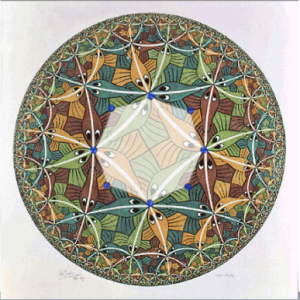Figure 8. Circle Limit III with the central tile of the octagonal tessellation , (polygon made using GeoGebra).

Circle Limit III
Circle Limit III is my favorite of the tessellations. In this work, the fish are nicely organized, their coloring properly alternates, and the images look more like real animals. This is achieved by alternating between triangles and quadrilaterals (as you can see from the defined white spines).

Although this image is more aesthetically pleasing, it did not pass the mathematical scrutiny of our very own Coxeter. As we discussed earlier, geodesic polygons must be made of perpendicular circle segments, but the arcs in Circle Limit III meet the boundary at about 80˚. An alternative view on the polygons, though, gives a true tessellation with octagons . The center polygon is indicated in Figure 8, and the rest of the tiles can be found in the same way, by connecting the nose vertices and the fin vertices. Notice that the edges of the polygon are not straight lines, and are instead segments of circles perpendicular to the boundary of the disc.

Circle Limit IV
Circle Limit IV (Figure 9) is the last piece in the series, and plays with negative space to alternate angels and devils. It is commonly referred to as “Heaven and Hell,” and creates a hexagonal tiling–can you find it? (Hint: look at toes and wing tips to find vertices). Check this link to see if you found it. Escher also made a similar Euclidean tiling: Notebook Pattern or Symmetry Work 45 (1941) .

Today, most geometers use computers to study and create tessellations and other geometrical phenomena. The fact the Escher created these using only a compass and ruler is nothing short of miraculous. (This  column explores the nuts and bolts of how he could have achieved that–an especially hard task considering he was working in wood cuts). And recall that Escher was working not only without the aide of technology, but also without the aide of a formal math education. Keeping that in mind, although the technicalities of non-Euclidean spaces can be daunting, the mathematical beauty that arises from hyperbolic geometry are within your grasp, too.

References

 Bonahon, Francis. Low-Dimensional Geometry: From Euclidean Surfaces to Hyperbolic Knots, American Mathematical Society, 2009.
 Casselman, Bill. How Did Escher Do It?, America Mathematical Society Feature Column.
 Dunham, Douglas. Transformation of Hyperbolic Escher Patterns, University of Minnesota-Duluth.
 Mackenzie, Dana. The Universe in Zero Words, Elwin Street Press, 2012.
 Math & the Art of MC Escher. Hyperbolic Geometry, EscherMath, 2016.
 Math Explorer Club. M. C. Escher and Hyperbolic Geometry, Cornell University, funded by the National Science Foundation.
 MC Escher, website.
 National Geographic. Nudibranchs Photo Gallery.
 Organic Facts. Health Benefits of Lettuce.
 Schwartz, Richard Evan. Mostly Surfaces, American Mathematical Society, 2011.

This entry was posted in Uncategorized. Bookmark the permalink.

• ## History of Hyperbolic Geometry

Sami was a student in the Fall 2016 course “Geometry of Surfaces” taught by Scott Taylor at Colby College. The essay has been lightly edited before being published here.

Introduction
This essay is an introduction to the history of hyperbolic geometry. Euclid, Gauss, Felix Klein and Henri Poincare all made major contribution to the field. We will discuss some of their influences in the following sections, starting with Euclid and his postulates that defined geometry. Then we will look at the effect of Gauss’s thoughts on Euclid’s parallel postulate through noneuclidean geometry. Later, Klein settled any doubt of noneuclidean consistency. Lastly, Poincare makes some notable contributions to solidifying hyperbolic geometry as an area of academic study.

Euclid’s Postulates
Euclidean geometry came from Euclid’s five postulates. It is the most intuitive geometry in that it is the way humans naturally think about the world. Nonetheless, there are a few other lesser-known, but equally important, geometries that also have many applications in the world and the universe. These “other” geometries come from Euclid’s fifth postulate: “If a straight line falling on two straight lines makes the interior angles on the same side less than two right angles the two straight lines if produced indefinitely meet on that side on which the angles [are] less than two right angles” . See Figure 1(A) below for an illustration of this. An equivalent way to express this is that the angle sum of a triangle is two right angles.

This is also called the parallel postulate because it is equivalent to the following statement: “if one draws a straight line and a point not on that line, there is only one unique line that is parallel to given line through the point” . See Figure 1(B) below.

Unlike the other postulates, the fifth is not obvious (to satisfy your curiosity, the rest of Euclid’s postulates are attached at the end of this essay). For centuries, mathematicians and amateurs alike attempted to prove that the fifth postulate is a consequence of the first four postulates and other established theorems . However, it turns out this postulate determines whether we are in euclidean or noneuclidean geometry .

The Birth of Hyperbolic Geometry
Over 2,000 years after Euclid, three mathematicians finally answered the question of the parallel postulate. Carl F. Gauss, Janos Bolyai, and N.I. Lobachevsky are considered the fathers of hyperbolic geometry. Gauss published little of his work on it because he, allegedly, feared disrespecting Euclid by disproving the parallel postulate. Yet, Gauss extended geometry to include what he coined “non-euclidean,” or the contradiction of the parallel postulate . Bolyai and Lobachevsky published their works on noneuclidean geometry independently but around the same time; however, their findings were not popularized until after 1862, when Gauss’s private letter to Schumacher about his “meditations” on hyperbolic geometry was published. With the work of these three mathematicians, the controversy of the parallel postulate was put to rest. It was definitive that without the parallel postulate, the remaining four postulates created a geometry that is equally consistent .

What is hyperbolic geometry?
Now that a brief history of the sources of hyperbolic geometry has been provided, we will define hyperbolic geometry. It has constant negative Gaussian curvature, which resembles a hyperboloid (See Figure 2). Note, that spherical geometry has constant positive curvature .

First, we will give a few different ways to visualize hyperbolic geometry, then we will explain how to measure distance in this space.

Visualization of Hyperbolic Geometry
A more natural way to think about hyperbolic geometry is through a crochet model as shown in Figure 3 below. This discovery by Daina Taimina in 1997 was a huge breakthrough for helping people understand hyperbolic geometry when she crocheted the hyperbolic plane. This was important because now people can touch and move the crocheted object to get a more intuitive understanding of what a straight line in hyperbolic space would look like.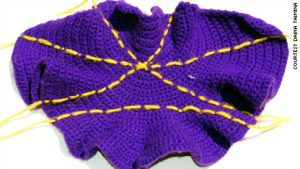Figure 3: A crochet from Daina Taimina . You can clearly see that the parallel postulate does not hold here because there are three lines that go through a point and none of them intersect with the given line on the bottom.

As mentioned before, we can visualize hyperbolic geometry through crochet. However, there are some different models including the upper-half plane and the Poincare disk model. See Figure 4 below. The upper-half plane model has the real line as the axis, which we may approach but will never reach. The disk model has the same idea as we approach the edge of the circle. Lines that appear to hit these ideal boundaries at the same point have an angle of 0 between them.

Definition of Distance
Although it may be a bit surprising at first, the definition of metric, or measure of distance, is different for each type of geometry. In Euclidean geometry, the distance is given as$d_{euc}= \sqrt{(x_1-x_0)^2+(y_1-y_0)^2}$

between two points$(x_0,y_0)$ and$(x_1,y_1)$. Note that in euclidean geometry, the shortest distance between two points is a line (as postulated by Euclid). However, in hyperbolic space, the shortest distance between two points is the arc from point$a$ to point$b$ that lie on the unique semicircle centered at a point on the boundary as shown in Figure 5; if we parameterize the arc as$t \mapsto (x(t),y(t)), \ \ a\leq t \leq b$,$l_{hyp} = \int_{a}^{b} \frac{\sqrt{x'(t)^2+y'(t)^2}}{y(t)} dt$

See .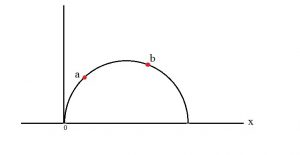Figure 5: Hyperbolic geodesic pictured as a euclidean semi-circle centered at a point on x.

Now that we’ve gone over the founding of hyperbolic geometry and have some sense of what it is, we’ll talk about two more influential mathematicians in the field: Felix Klein and Henri Poincare.

Felix Klein
Klein’s contribution to geometry is not only the famous Klein Bottle, but also his proof of the extension of the Cayley Measure on projective geometry to non-euclidean geometry. According to John Stillwell’s translation of On so-called Noneuclidean Geometry, Klein started by showing that each non-euclidean geometry is a special case of projective geometry; he then ended by concluding that non-euclidean geometry can be derived from projective geometry. Furthermore, projective geometry is independent from the theory of parallels; if not, the consistency of projective geometry would be questioned.

Projective Geometry

Projective geometry has simple origins from Renaissance artists who portrayed the rim of a cup as an ellipse in their paintings to show perspective. Projective geometry can be thought of in this way:

The theory of perspective regards the artist’s canvas as a transparent screen through which he looks from a fixed vantage point at the scene he is painting. Light rays coming from each point of the scene are imagined to enter his eye, and the totality of these lines is called a projection. 

From there, the idea eventually entered the academic mathematical community where it was incorporated into the study of linear algebra and other areas of mathematics. Projective geometry is the study of invariants on projections – properties of figures which are not modified in the process of projection . Projective geometry is more more general than both euclidean and hyperbolic geometries and this is what Klein uses to show that noneuclidean geometries are consistent.

Euclidean and hyperbolic geometry follows from projective geometry

Klein gives a general method of constructing length and angles in projective geometry, which he believed to be the fundamental concept of geometry. To borrow psychology terms, Klein’s approach is a top-down way to look at non-euclidean geometry while the upper-half plane, disk model and other models would be referred to as bottom-up methods. The bottom-up methods are easier to visualize and to deal with applications of hyperbolic geometry. This is remarkable because this top-down approach provides a “more comprehensive”  proof than those that had in a case by case manner. Moreover, projective geometry is consistent outside of geometry .

Distance in general
The different geometries we get from projective geometry come from the the projection of the fundamental conic. This idea is illustrated below in Figure 7.

First, Klein defines the general distance function as$2ic \text{ arccos }\frac{\Omega_{xy}}{\sqrt{\Omega_{xx} \Omega_{yy}}}$
where the constant$c$ determines which space we are in (Hyperbolic, parabolic, elliptic) and$\begin{array}{rcl}\Omega_{xx} &=& ax_1 ^2+bx_1x_2+cx_2 ^2\\ \Omega_{yy} &=& ay_1 ^2+by_1y_2+cy_2 ^2 \ \text{and} \\ \Omega_{xy} &=& ax_1y_1+b(x_1y_2+y_1x_2)+cx_2y_2 \end{array}$
with$(x_1,x_2) \text{ and } (y_1,y_2)$ as the coordinates of two points.

Klein shows that the fundamental conic section “corresponds completely with the idea of hyperbolic geometry.” Now, the fundamental conic is the projection onto the plane with the appropriate metric; it “determines a measure on all basic figures of the first kind in the plane”. Here, the “first kind” is fuchsian groups , which are isometries of the hyperbolic plane. An isometry is a way of transforming a figure in a given space without changing its angles or lengths. Every isometry in hyperbolic space can be written as a linear fractional transformation in the following form:$\phi(z) = \frac{az+b}{cz+d}$

where$z$ is the complex coordinate of a point. You can think of a complex coordinate in the same way that you think about$(x,y)$ on the euclidean plane where$z = x +\text{i}y$. The “basic figures” are the triangle, circle, and the square. So these isometries take triangles to triangles, circles to circles and squares to squares.

The fundamental conic that forms hyperbolic geometry is proper and real – but “we shall never reach the fundamental conic, let alone pass beyond it”. Recall, our visualizations of hyperbolic space using the upper-half plane model from Figure 4(A), then the fundamental conic is the real line and the fuchsian groups are the isometries acting on$\mathbb{H}^2$.

By using projective geometry to construct noneuclidean geometries, Klein has created a proof that is indisputable.

Henri Poincare
A discussion of the history of hyperbolic geometry cannot leave out Henri Poincare after which the Poincare disk model is named (See Figure 4 (B)). Originally, he began his studies in differential equations, then stumbled upon hyperbolic geometry naturally in his work. He wrote in his book Science and Methods that:

The incidents of travel made me forget my mathematical work. When we arrived at Countances, we got into a break to go for a drive, and, just as I put my foot on the step, the idea came to me, though nothing in my former thoughts seemed to have prepared me for it, that the transformations I had used to define Fuchsian functions were identical with those of non-euclidean geometry. 

In fact, he was studying the differential equation$x''(t)+p(t)x(t)=0$ for a given function$p(t)$ that resembles linear fractional transformations with real coefficients. When perturbed to have slightly complex coefficients, this yielded a group$\Gamma$ of linear fractional maps with complex coefficients that act on the Riemann Sphere. This is also related to chaos! He called the ones with real coefficients fuchsian groups after Fuchs and the ones with complex coefficients kleinian groups, though neither Fuchs nor Klein had much to do with the groups they are named after. Allegedly, Klein was offended when Poincare declared the fuchsian group, claiming it had been he, not Fuchs, who had thought about it. So to appease Klein, Poincare named the next group after him. Nonetheless, these are groups of isometries on the hyperbolic plane,$\mathbb{H}^2$ .

There have been many other theorems (and still more though current research) in hyperbolic space but these have been instrumental in the creation of hyperbolic geometry. Despite its turbulent history surrounding the parallel postulate and its late debut in the mathematical world, hyperbolic geometry is gaining prominence and has many great applications to the real world.

Euclid’s Postulates :

1. A straight line segment can be drawn joining any two points.
2. Any straight line segment can be extended indefinitely in a straight line.
3. Given any straight lines segment, a circle can be drawn having the segment as radius and one endpoint as center.
4. All Right Angles are congruent.
5. If two lines are drawn which intersect a third in such a way that the sum of the inner angles on one side is less than two Right Angles, then the two lines inevitably must intersect each other on that side if extended far enough.

References:

 Antonick, Gary. “The Non-Euclidean Geometry of Whales.” The New York Times. The New York Times, 08 Oct. 2012. Web. 04 Dec. 2016.
 Bonahon, Francis. Low-dimensional Geometry: From Euclidean Surfaces to Hyperbolic Knots. Providence, RI: American Mathematical Society, 2009. Print.
 Creates, Cris. “Crochet Mathematics: Hyperbolic Crochet.” Crochet Mathematics: Hyperbolic Crochet. N.p., 12 Jan. 2012. Web. 04 Dec. 2016.
 “Daina Taimina.” Daina Taimina | Crochet Coral Reef. N.p., n.d. Web. 04 Dec. 2016.
 Henderson, David W. Experiencing Geometry: In Euclidean, Spherical, and Hyperbolic Spaces. Contributer: Daina Taimina. 2nd ed. Upper Saddle River, NJ: Prentice Hall, 2001. Print.
 Lewis, Florence P. “History of the Parallel Postulate.” The American Mathematical Monthly, vol. 27, no. 1, 1920, pp. 16–23. www.jstor.org/stable/2973238.
 McMullen, Curtis T. “Euclid’s Postulates.” Euclid’s Postulates. Harvard University, 1997. Web. 07 Dec. 2016.
 Nicoguaro. Gaussian Curvature. Digital image. Wikicommons, 9 Jan. 2016. Web. 6 Dec. 2016.
 Poincaré, Henri. Science and Methods. Trans. Francis Maitland. London: T Nelson, 1914. Archive.org.
 Stillwell, John. “Introduction and Translation to Klein’s On the So-called Noneuclidean Geometry.” Sources of Hyperbolic Geometry. Vol. 10. Providence, RI: American Mathematical Society, 1996. 63-111. Print. History of Mathematics.
 Wylie, C. R. “Introduction to Projective Geometry.” Google Books. Courier Corporation, 2011. Web. 08 Dec. 2016.

This entry was posted in Hyperbolic Geometry and tagged , , . Bookmark the permalink.